# GCSE Maths Paper 01 (Foundation) Practice Paper in the Style of OCR - Calculator

In this assessment, students will be able to complete a timed GCSE Mathematics Paper 01 (Calculator) in the style of OCR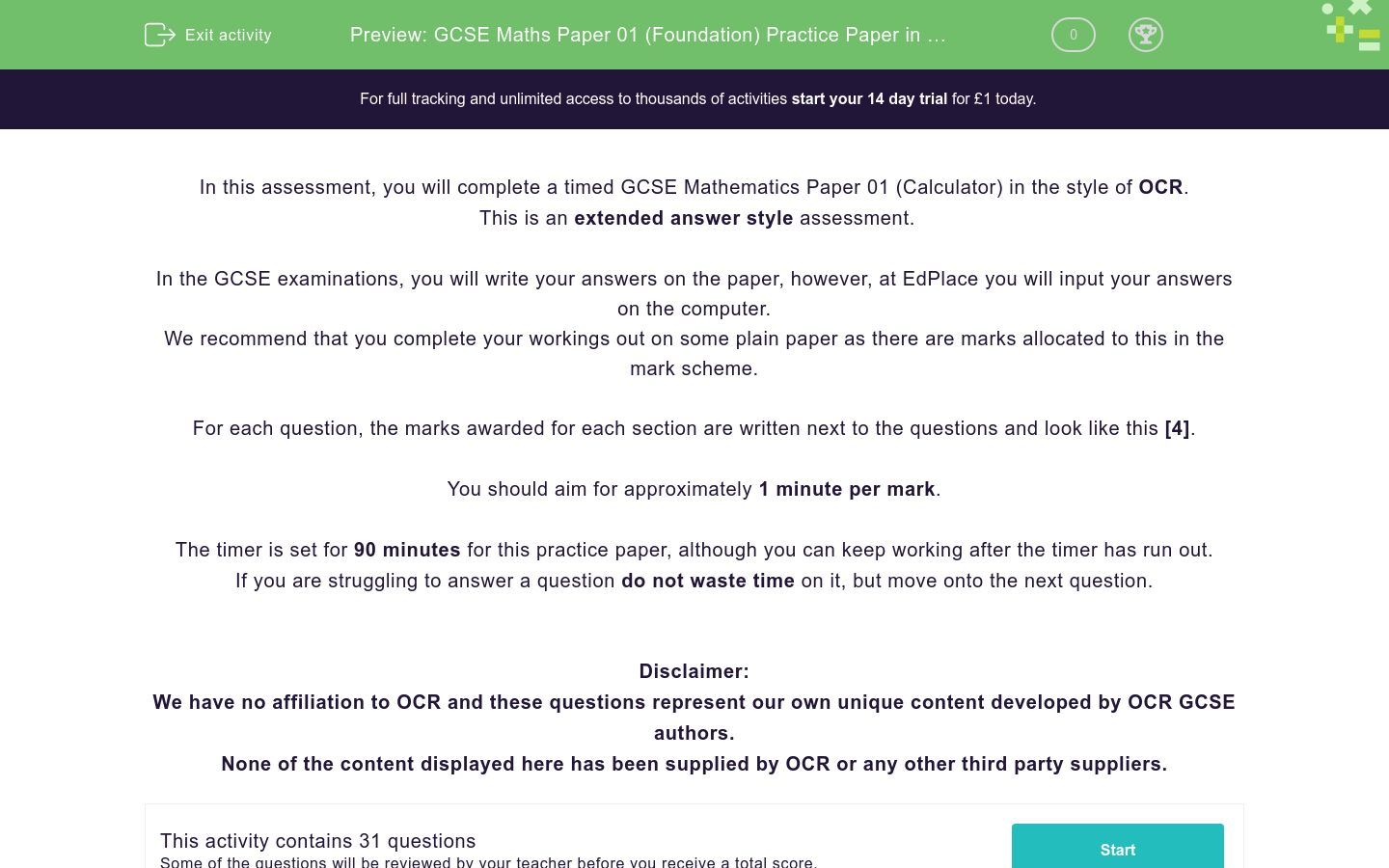Key stage:  KS 4

GCSE Subjects:   Maths

GCSE Boards:   OCR

Curriculum topic:   Foundation Practice Papers

Curriculum subtopic:   Calculator Practice Papers

Difficulty level:### QUESTION 1 of 10

In this assessment, you will complete a timed GCSE Mathematics Paper 01 (Calculator) in the style of OCR.

This is an extended answer style assessment.

In the GCSE examinations, you will write your answers on the paper, however, at EdPlace you will input your answers on the computer.

We recommend that you complete your workings out on some plain paper as there are marks allocated to this in the mark scheme.

For each question, the marks awarded for each section are written next to the questions and look like this .

You should aim for approximately 1 minute per mark.

The timer is set for 90 minutes for this practice paper, although you can keep working after the timer has run out.

If you are struggling to answer a question do not waste time on it, but move onto the next question.

Disclaimer:

We have no affiliation to OCR and these questions represent our own unique content developed by OCR GCSE authors.

None of the content displayed here has been supplied by OCR or any other third party suppliers.

Write 0.65 as a fraction



Write the following numbers in order of size

-2          2          -1          0            3



Write down 2 factors of 24



Change 1.23 km into metres.



Write the number sixty-five thousand in figures.



Derek is thinking of a number.

He says…

“My number is even. It is a factor of 40 and a multiple of 5”

There are 3 numbers that he could be thinking of, write down at least two of them.



Amrit says.

“There are more square numbers between 1 and 10 than there are between 11 and 30.”

Is Amrit right?



A map has a scale of 1 cm to 25 km

On the map, the distance between Manchester and Edinburgh is 11.2 cm.

What is the real distance, in km, between Manchester and Edinburgh?



The angles, in degrees, of this rectangle, are shown as algebraic expressions.Find the value of x.



Here is a rule.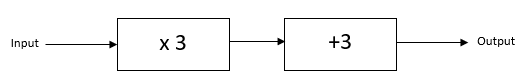(a) Work out the output when the input is 4

(b) Work out the input when the output is 6



Solve…

(a) 2a + a + 3a = 54

(b) b/3 = 6

(c) 3c – 2 = 16.6



Here is part of a train timetable

 Leeds 07:05 07:24 07:41 Manchester 07:56 08:11 08:58

Kate gets to the train station at Leeds at 07:09

(a) Work out how many minutes she has to wait to get the first train.

(b) Work out the time taken for the 07:41 train to get to Manchester.



James washes cars.

He gets paid 59 p per car and washes an average of 12 cars an hour.

Jim works in a call centre. He gets paid £269.80 for a 38 hour week.

Show that Jim has a higher hourly pay than James.



x and y are even numbers

(a) Give an example to show that 3(2x – y) is a multiple of 6

(b) Show that when x and y are both even, the value of 3 (2x – y) will always be a multiple of 6.



A garden bed is in the shape of a rectangle 2m by 3m

Flowers are grown in 47% of the bed.

Vegetables are grown in the rest of the bed.

Work out the area of the garden that is used to grow vegetables.



Solve 3x + 6 = 2(4x – 3)



Here are the ingredients for making 10 sconesJohn has the following ingredients.

1.2 kg flour

A full tin of baking powder

150 g of caster sugar

250 g of butter

What is the greatest number of scones that John can make?



There are 78 calories in 100g of cereal

There are 142 calories in 200 ml of milk

Alexa has 150 g of cereal and 250 ml of milk for her breakfast.

How many calories are there in this meal?



Machine 1 and Machine 2 both make parts for motorbikes

Machine 1 makes 10 parts every 15 minutes

Machine 2 makes 14 parts every 20 minutes

Both machines run for 8 hours per day, Monday – Friday.

How many parts can the machines make in 1 week?



The table shows the probability that a biased dice will land on 1, on 2, on 3, on 4 and on 6.

 Number 1 2 3 4 5 6 Probability 0.3 0.05 0.12 0.13 0.21

Gemma rolls the dice 150 times. Estimate the total number of times the dice will land on a prime number.



Some adults and children went to see a rugby match.

The ratio of children to adults was 2:7

Each person either had a seated or a standing ticket.

4/5 of the children had seated tickets

The stadium has a capacity of 9500.

Was the stadium more than 53% full?



BEG is a triangle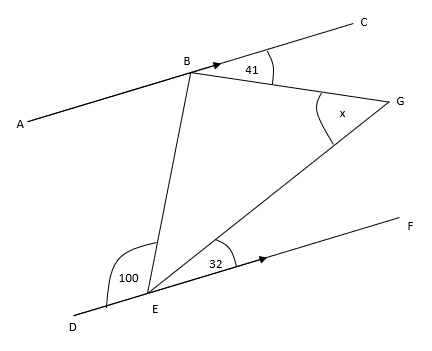ABC and DEF are parallel lines

Work out the size of angle x giving reasons for each stage of your working.



There are only red, black and blue marbles in a bag.

There are three times as many black marbles as red marbles and twice as many blue marbles as black.

Amelia takes a marble from the bag at random.

Find the probability it is a black marble.ABD and ACE are straight lines

BC is parallel to DB

AB = 4.2 cm

DE = 9 cm

(a) Work out the length of BC

AE = 7.74 cm

(b) Work out the length of CE



A force of 80 newtons acts on an area of 15 cm2.The force is increased by 5 newtons

The area is decreased by 2 cm2.

Javed says;

“The pressure decreases by less than 10%.”

Is Javed correct?



(a) Write the number 0.005648 in standard form.

(b) Work out100 members of a triathlon club were asked if their favourite sport was cycling, swimming or running.

52 of the people asked were male

12 of the 41 who said cycling were female

19 men said running

17 people said swimming.

One of the female members is picked at random, what is the probability she said swimming was her favourite sport?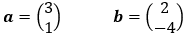Work out 2a + 3b as a column vector.



(a) Write 15:60 as a ratio in its simplest form.     

(b) The ratio 40p:£2.50 can be written in the form 1:n.

Find the value of n.                    

(c) James and David share some money in the ratio 3: 5.

Work out how much money was shared.           

Triangle R is drawn on a coordinate grid.(a) Rotate triangle R through 180° about (0,0)

(b) Reflect triangle R in the line y = - 1.

The table below show the weight, w kg, of 100 people.

 Weight (w kg) Frequency 40 < w ≤ 60 5 60 < w ≤ 80 9 80 < w ≤ 100 22 100 < w ≤ 120 27 120 < w ≤ 140 26 140 < w ≤ 160 11

Find an estimate of the mean weight in kilograms.



• Question 1

Write 0.65 as a fraction



65/100
13/20
EDDIE SAYS
When you need to convert decimals into fractions, the trick is to look at the last digit. In this case the 5 is in the hundredths column. This gives a fraction of 65/100, all you need to do is cancel it down.
• Question 2

Write the following numbers in order of size

-2          2          -1          0            3



EDDIE SAYS
Look at the number line, all you are doing is going left to right. Remember that, for example, -3 is smaller than -2
• Question 3

Write down 2 factors of 24



EDDIE SAYS
The factors of 24 are just the numbers that you can divide by without leaving a remainder. So 24 &div: 8 = 3 (3 and 8 are factors of 24) but 24 ÷7 = 3.429 so 7 is not a factor of 24
• Question 4

Change 1.23 km into metres.



1230
1230m
1230 m
EDDIE SAYS
All you need to know here is that the conversion factor for m into km is 1000 Because there is more metres than km in the same distance, we have to multiply by 1000
• Question 5

Write the number sixty-five thousand in figures.



65000
65,000
EDDIE SAYS
Look at your place values here: Sixty thousand = 60000 Five thousand = 5000 Just add them together.
• Question 6

Derek is thinking of a number.

He says…

“My number is even. It is a factor of 40 and a multiple of 5”

There are 3 numbers that he could be thinking of, write down at least two of them.



EDDIE SAYS• Question 7

Amrit says.

“There are more square numbers between 1 and 10 than there are between 11 and 30.”

Is Amrit right?



EDDIE SAYS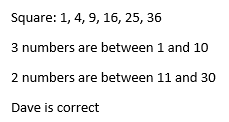• Question 8

A map has a scale of 1 cm to 25 km

On the map, the distance between Manchester and Edinburgh is 11.2 cm.

What is the real distance, in km, between Manchester and Edinburgh?



EDDIE SAYS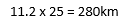• Question 9

The angles, in degrees, of this rectangle, are shown as algebraic expressions.Find the value of x.



EDDIE SAYS• Question 10

Here is a rule.(a) Work out the output when the input is 4

(b) Work out the input when the output is 6



EDDIE SAYS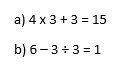• Question 11

Solve…

(a) 2a + a + 3a = 54

(b) b/3 = 6

(c) 3c – 2 = 16.6



EDDIE SAYS• Question 12

Here is part of a train timetable

 Leeds 07:05 07:24 07:41 Manchester 07:56 08:11 08:58

Kate gets to the train station at Leeds at 07:09

(a) Work out how many minutes she has to wait to get the first train.

(b) Work out the time taken for the 07:41 train to get to Manchester.



EDDIE SAYS• Question 13

James washes cars.

He gets paid 59 p per car and washes an average of 12 cars an hour.

Jim works in a call centre. He gets paid £269.80 for a 38 hour week.

Show that Jim has a higher hourly pay than James.



EDDIE SAYS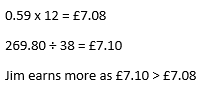• Question 14

x and y are even numbers

(a) Give an example to show that 3(2x – y) is a multiple of 6

(b) Show that when x and y are both even, the value of 3 (2x – y) will always be a multiple of 6.



EDDIE SAYS• Question 15

A garden bed is in the shape of a rectangle 2m by 3m

Flowers are grown in 47% of the bed.

Vegetables are grown in the rest of the bed.

Work out the area of the garden that is used to grow vegetables.



EDDIE SAYS• Question 16

Solve 3x + 6 = 2(4x – 3)



EDDIE SAYS• Question 17

Here are the ingredients for making 10 sconesJohn has the following ingredients.

1.2 kg flour

A full tin of baking powder

150 g of caster sugar

250 g of butter

What is the greatest number of scones that John can make?



EDDIE SAYS• Question 18

There are 78 calories in 100g of cereal

There are 142 calories in 200 ml of milk

Alexa has 150 g of cereal and 250 ml of milk for her breakfast.

How many calories are there in this meal?



EDDIE SAYS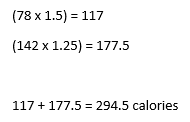• Question 19

Machine 1 and Machine 2 both make parts for motorbikes

Machine 1 makes 10 parts every 15 minutes

Machine 2 makes 14 parts every 20 minutes

Both machines run for 8 hours per day, Monday – Friday.

How many parts can the machines make in 1 week?



EDDIE SAYS• Question 20

The table shows the probability that a biased dice will land on 1, on 2, on 3, on 4 and on 6.

 Number 1 2 3 4 5 6 Probability 0.3 0.05 0.12 0.13 0.21

Gemma rolls the dice 150 times. Estimate the total number of times the dice will land on a prime number.



EDDIE SAYS• Question 21

Some adults and children went to see a rugby match.

The ratio of children to adults was 2:7

Each person either had a seated or a standing ticket.

4/5 of the children had seated tickets

The stadium has a capacity of 9500.

Was the stadium more than 53% full?



EDDIE SAYS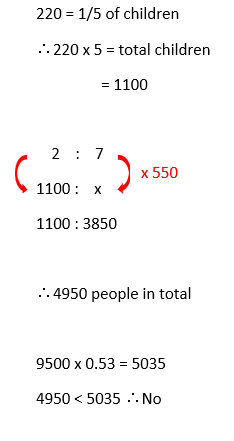• Question 22

BEG is a triangleABC and DEF are parallel lines

Work out the size of angle x giving reasons for each stage of your working.



EDDIE SAYS• Question 23

There are only red, black and blue marbles in a bag.

There are three times as many black marbles as red marbles and twice as many blue marbles as black.

Amelia takes a marble from the bag at random.

Find the probability it is a black marble.



EDDIE SAYS• Question 24ABD and ACE are straight lines

BC is parallel to DB

AB = 4.2 cm

DE = 9 cm

(a) Work out the length of BC

AE = 7.74 cm

(b) Work out the length of CE



EDDIE SAYS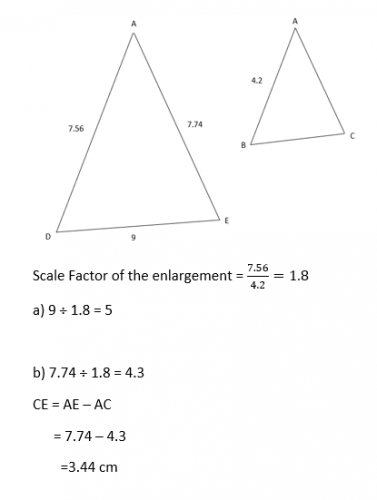• Question 25

A force of 80 newtons acts on an area of 15 cm2.The force is increased by 5 newtons

The area is decreased by 2 cm2.

Javed says;

“The pressure decreases by less than 10%.”

Is Javed correct?



EDDIE SAYS• Question 26

(a) Write the number 0.005648 in standard form.

(b) Work outEDDIE SAYS• Question 27

100 members of a triathlon club were asked if their favourite sport was cycling, swimming or running.

52 of the people asked were male

12 of the 41 who said cycling were female

19 men said running

17 people said swimming.

One of the female members is picked at random, what is the probability she said swimming was her favourite sport?



EDDIE SAYS• Question 28Work out 2a + 3b as a column vector.



EDDIE SAYS• Question 29

(a) Write 15:60 as a ratio in its simplest form.     

(b) The ratio 40p:£2.50 can be written in the form 1:n.

Find the value of n.                    

(c) James and David share some money in the ratio 3: 5.

Work out how much money was shared.           

EDDIE SAYS• Question 30

Triangle R is drawn on a coordinate grid.(a) Rotate triangle R through 180° about (0,0)

(b) Reflect triangle R in the line y = - 1.

EDDIE SAYS• Question 31

The table below show the weight, w kg, of 100 people.

 Weight (w kg) Frequency 40 < w ≤ 60 5 60 < w ≤ 80 9 80 < w ≤ 100 22 100 < w ≤ 120 27 120 < w ≤ 140 26 140 < w ≤ 160 11

Find an estimate of the mean weight in kilograms.



EDDIE SAYS